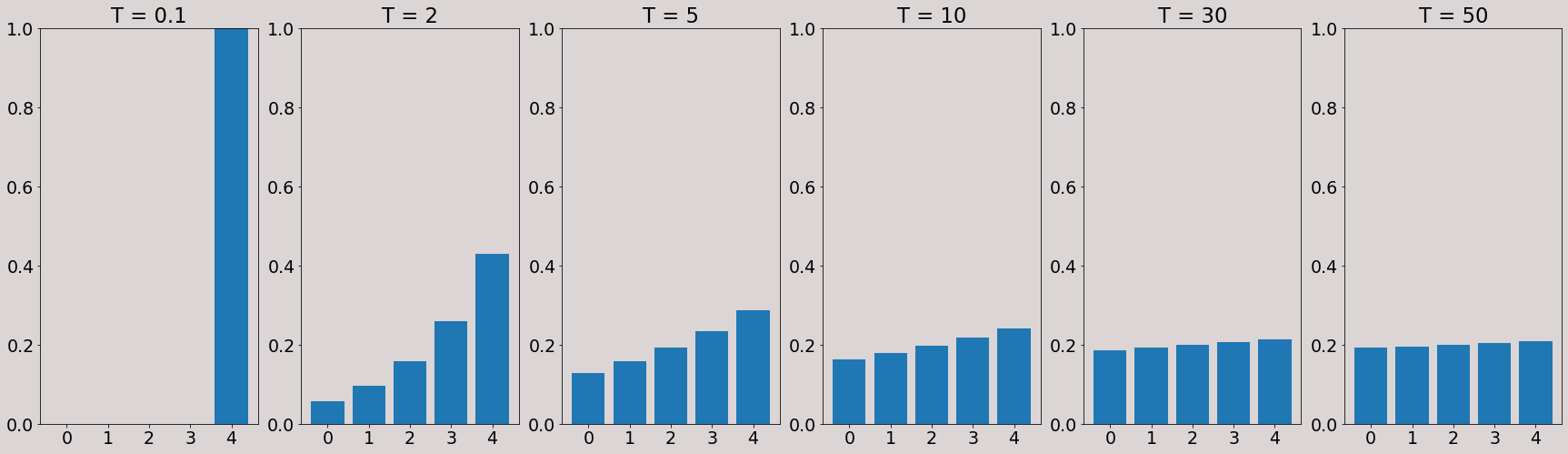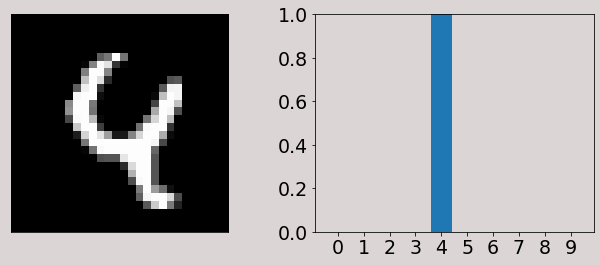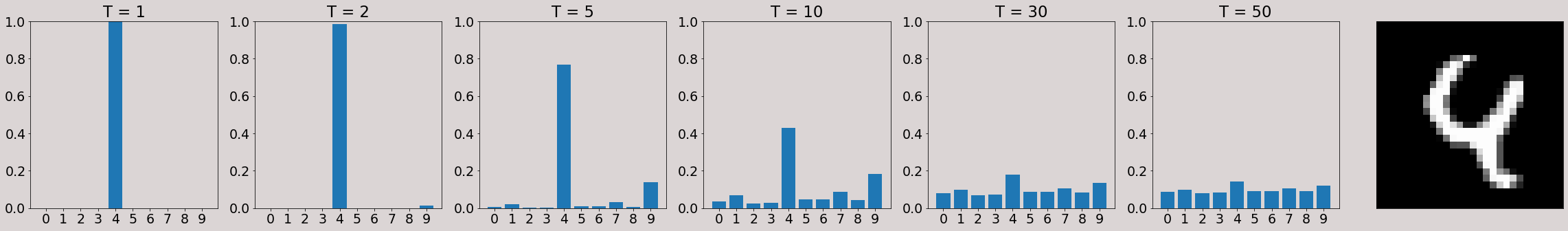# Introduction to Knowledge Distillation

Published Oct 22, 2022 by Aditya Mehrotra

This post will go over the math and mechanics of how Knowledge Distillation works and also include some code on how to implement it.

## Preliminaries:

Say you have a classification task, where you have some feedforward net to classify the digits of MNIST. Denote the number of samples in the minibatch as $m$, so we index a sample with $i \in [1, m]$ and also denote the number of output classes as $n \in \mathbb{N}$. The feedforward net produces unnormalized class scores (final output from the model), which we will denote as a vector $z_i \in \mathbb{R}^n$. Finally, we retrieve a probability distribution over the output classes using the softmax function applied to the $z_i$, we will denote this as $a_i \in \mathbb{R}^n$. Finally, I will use an arbitrary $k \in [0, n-1]$ to index my vectors. So $z_{i,k}$ represents the $k^{th}$ element of $z_i$, and likewise for $a_i$. Here is the formula:

$$\LARGE{a_{i,k} := \frac{e^{z_{i,k}}}{\sum_{j=0}^{n-1} e^{z_{i,j}}}}$$

Here is an example of it being used (rounded to four digits):

\begin{align} \small{\begin{bmatrix} 2 \\ 10 \\ 3 \\ 0 \\ 5 \\ 4 \\ 7 \\ 9 \\ 1 \\ 2 \end{bmatrix}} \Longrightarrow softmax\left(\begin{bmatrix} 2 \\ 10 \\ 3 \\ 0 \\ 5 \\ 4 \\ 7 \\ 9 \\ 1 \\ 2 \end{bmatrix}\right) \Longrightarrow \begin{bmatrix} 0.0002 \\ 0.7000 \\ 0.0006 \\ 0.0000 \\ 0.0047 \\ 0.0017 \\ 0.0348 \\ 0.2575 \\ 0.0001 \\ 0.0002 \end{bmatrix} \end{align}

The ground truth one hot vector is denoted $y_i$ for sample $i$ and the output softmax distribution is denote $\hat{y_i} = a_i$. The loss function that is traditionally used is categorical cross entropy loss. Also, assume your ground truth labels are some one hot encoded vector, with a one at the index of the true class label. Here is its form with a few simplifications:

$$CE(y, \hat{y}) = -\frac{1}{m} \sum_{i=1}^{m} \sum_{j=0}^{n-1} y^j \cdot log(\hat{y_i})$$ $$= -\frac{1}{m} \sum_i^{m}log(\hat{y_i})$$

Intuitively, for each datapoint, we are trying to maximize the log probability of the GT class, disregarding the probabilities of the other classes.

## Softmax Temperature:

Now, let us modify our softmax function a little bit:

$$\Large{a_{i,k} := \frac{e^{\frac{z_{i,k}}{T}}}{\displaystyle\sum_{j=1}^{n-1} e^{\frac{z_{i,j}}{T}}}}$$

I have introduced a new hyperparameter $T$, which is commonly called "temperature" or "softmax temperature". Notice that if $T = 1$, we simply have our old softmax expression. Firstly, convince yourself that as $T \rightarrow \infty$, each $a_{i,k}$ will approach $\frac{1}{n}$. This means that as $T$ gets larger, the softmax distribution becomes a more softer probability distribution over the classes. Here are a few examples where $n=5$ and $z_{i} = [1,2,3,4,5]$:As you can see, the distribution gets softer and softer peaks as we increase $T$ and generally seems to be approaching a uniform distribution. However, relationship between the class probabilities with regards to size stays about the same. As shown above, the classes 0-4 have increasing probability from right to left, except for very high values of $T$.

## "Dark Knowledge":

Now here's the interesting bit, assume we trained a simple feedforward classifier on MNIST ($n = 10$). If we sample the following image and feed it into the classifier, we get the following softmax scores. $T$ is set to 1, so this is just with the standard softmax function.Pretty good right? Notice that the probability for the GT class 4 is much higher than the others, so our distribution peaks very highly at a certain point. The other probabilities are very small in comparison, and are not really interpertable. Now lets turn up the temperature to some higher values of $T$.The distribution is getting softer and softer as we increase the temperature, and we begin to see the relationship between the smaller probabilities. The ground truth class is a 4, but the image also looks a lot like a 9. The classifier's softmax distribution has a high probability given to 9 for temperature values of 5 and 10 compared to the other classes. This is what we mean by "dark knowledge". By increasing the temperature, we reveal information about what other likely classes the image can belong to. We call these probability distributions generated through softmax with temperature "soft labels". Soft labels are a lot more meaningful than the standard one hot encoded labels since they encode information about what classes the sample resembles. This boosts its ability to classify other classes that aren't the ground truth class.

## Aside: Why is there a meaningful relationship between probabilities of non-ground truth classes in a softmax distribution?

A core reason of why knowledge distillation works is because we assume that the softmax probabilities of classes that aren't the ground truth class are meaningful. Specifically in the knowledge distillation paper, Hinton et al. state that:

"Much of the information about the learned function resides in the ratios of very small probabilities in the soft targets. For example, one version of a 2 may be given a probability of $10^6$ of being a 3 and $10^9$ of being a 7 whereas for another version it may be the other way around. This is valuable information that defines a rich similarity structure over the data (i. e. it says which 2’s look like 3’s and which look like 7’s)"

However, I wasn't too sure what is the reasoning behind why we can say this and had the following question:

Couldn't the model learn to give a high probability to the target class for an image and meaningless assorted probabilities to the others?

After struggling with this question for a while, I found a satisfying answer from asking around on r/learnmachinelearning . Here is the short version: First, we adopt the view that the model is learning to identify some high-level features from a given sample, where the final softmaxed scores say "How much do this sample's features resemble what samples from class X would look like?". Now, in datasets there is usually overlap between samples, even samples from different classes! Therefore, due to this overlap, it is evident that each of the class probabilities should contain information about how much the sample resembles class X, rather than the non-ground-truth probabilities being meaningless noise.

## Distillation loss:

Given a model trained on a dataset using the standard softmax activation (when $T=1$), which we call a teacher model, we want to train another model (potentially with a different architecture) called the student model.

Denote the logits of student network as $z_i$ and denote the logits of the teacher network as $\tilde z_i$. The student model is trained using the following objective, with a fixed hyperparameter $T$:

$$L = \alpha \cdot CE(y_i, \text{S}(z_i)) +\\ (1- \alpha) \cdot CE(\text{S}(\frac{z_i}{T}), \text{S}(\frac{\tilde z_i}{T}))$$

Where $S$ is the softmax function.

As you can see, we are trying to get the student model to maximize both the probability of the ground truth class through the first term. Additionally, we are also trying to match the distribution of the student softmax to the distribution of the teacher softmax, both with temperature $T$. We can say this because of the connections between cross entropy and KL divergence.

## Putting it together:

I will go over the steps required to distill knowledge from a teacher network into a (perhaps smaller) student network.

1. First, train a teacher network as you would normally, the final activation on the logits needs to be a softmax. Save the weights.
2. Train a student network, another classifier with a softmax activation, using the previously explanied loss

Thank you for reading this post!

I've implemented all of the concepts I've talked about here, you can find my code here. I plan on updating this post with my experimental results (and some particularily interesting ones around learning unseen classes) at a later date.